BRAIN TEASERS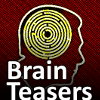# Daily Brain Teasers

puzzles, riddles, mathematical problems, word games, mastermind, cinemania, music, stereograms, ...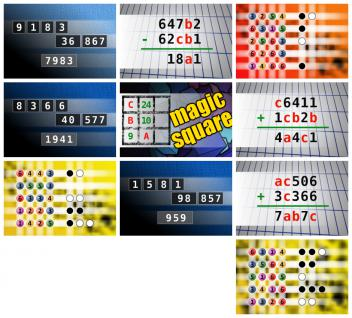Daily Brain Teasers for Monday, 02 October 2023
● NUMBERMANIA: Calculate the number 7983 using numbers [9, 1, 8, 3, 36, 867] and basic arithmetic operations (+, -, *, /). Each of the numbers can be used only once. ● If 647b2 - 62cb1 = 18a1 find number abc. Multiple solutions may exist. ● The computer chose a secret code (sequence of 4 digits from 1 to 6). Your goal is to find that code. Black circles indicate the number of hits on the right spot. White circles indicate the number of hits on the wrong spot. ● NUMBERMANIA: Calculate the number 1941 using numbers [8, 3, 6, 6, 40, 577] and basic arithmetic operations (+, -, *, /). Each of the numbers can be used only once. ● The aim is to place the some numbers from the list (9, 10, 13, 14, 15, 18, 24, 25, 28, 62) into the empty squares and squares marked with A, B an C. Sum of each row and column should be equal. All the numbers of the magic square must be different. Find values for A, B, and C. Solution is A+B+C. ● If c6411 + 1cb2b = 4a4c1 find number abc. Multiple solutions may exist. ● The computer chose a secret code (sequence of 4 digits from 1 to 6). Your goal is to find that code. Black circles indicate the number of hits on the right spot. White circles indicate the number of hits on the wrong spot. ● NUMBERMANIA: Calculate the number 959 using numbers [1, 5, 8, 1, 98, 857] and basic arithmetic operations (+, -, *, /). Each of the numbers can be used only once. ● If ac506 + 3c366 = 7ab7c find number abc. Multiple solutions may exist. ● The computer chose a secret code (sequence of 4 digits from 1 to 6). Your goal is to find that code. Black circles indicate the number of hits on the right spot. White circles indicate the number of hits on the wrong spot.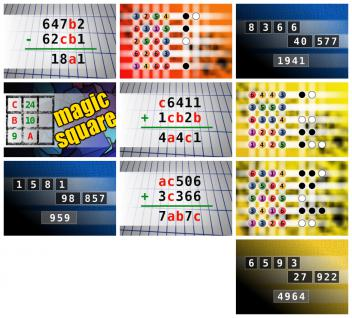Daily Brain Teasers for Sunday, 01 October 2023
● If 647b2 - 62cb1 = 18a1 find number abc. Multiple solutions may exist. ● The computer chose a secret code (sequence of 4 digits from 1 to 6). Your goal is to find that code. Black circles indicate the number of hits on the right spot. White circles indicate the number of hits on the wrong spot. ● NUMBERMANIA: Calculate the number 1941 using numbers [8, 3, 6, 6, 40, 577] and basic arithmetic operations (+, -, *, /). Each of the numbers can be used only once. ● The aim is to place the some numbers from the list (9, 10, 13, 14, 15, 18, 24, 25, 28, 62) into the empty squares and squares marked with A, B an C. Sum of each row and column should be equal. All the numbers of the magic square must be different. Find values for A, B, and C. Solution is A+B+C. ● If c6411 + 1cb2b = 4a4c1 find number abc. Multiple solutions may exist. ● The computer chose a secret code (sequence of 4 digits from 1 to 6). Your goal is to find that code. Black circles indicate the number of hits on the right spot. White circles indicate the number of hits on the wrong spot. ● NUMBERMANIA: Calculate the number 959 using numbers [1, 5, 8, 1, 98, 857] and basic arithmetic operations (+, -, *, /). Each of the numbers can be used only once. ● If ac506 + 3c366 = 7ab7c find number abc. Multiple solutions may exist. ● The computer chose a secret code (sequence of 4 digits from 1 to 6). Your goal is to find that code. Black circles indicate the number of hits on the right spot. White circles indicate the number of hits on the wrong spot. ● NUMBERMANIA: Calculate the number 4964 using numbers [6, 5, 9, 3, 27, 922] and basic arithmetic operations (+, -, *, /). Each of the numbers can be used only once.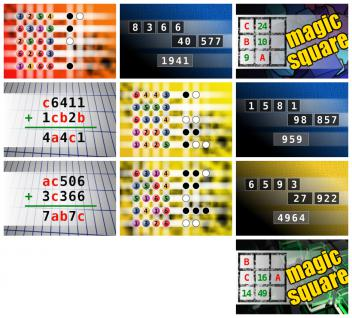Daily Brain Teasers for Saturday, 30 September 2023
● The computer chose a secret code (sequence of 4 digits from 1 to 6). Your goal is to find that code. Black circles indicate the number of hits on the right spot. White circles indicate the number of hits on the wrong spot. ● NUMBERMANIA: Calculate the number 1941 using numbers [8, 3, 6, 6, 40, 577] and basic arithmetic operations (+, -, *, /). Each of the numbers can be used only once. ● The aim is to place the some numbers from the list (9, 10, 13, 14, 15, 18, 24, 25, 28, 62) into the empty squares and squares marked with A, B an C. Sum of each row and column should be equal. All the numbers of the magic square must be different. Find values for A, B, and C. Solution is A+B+C. ● If c6411 + 1cb2b = 4a4c1 find number abc. Multiple solutions may exist. ● The computer chose a secret code (sequence of 4 digits from 1 to 6). Your goal is to find that code. Black circles indicate the number of hits on the right spot. White circles indicate the number of hits on the wrong spot. ● NUMBERMANIA: Calculate the number 959 using numbers [1, 5, 8, 1, 98, 857] and basic arithmetic operations (+, -, *, /). Each of the numbers can be used only once. ● If ac506 + 3c366 = 7ab7c find number abc. Multiple solutions may exist. ● The computer chose a secret code (sequence of 4 digits from 1 to 6). Your goal is to find that code. Black circles indicate the number of hits on the right spot. White circles indicate the number of hits on the wrong spot. ● NUMBERMANIA: Calculate the number 4964 using numbers [6, 5, 9, 3, 27, 922] and basic arithmetic operations (+, -, *, /). Each of the numbers can be used only once. ● The aim is to place the some numbers from the list (13, 14, 16, 20, 21, 23, 42, 49, 50, 52, 58, 79) into the empty squares and squares marked with A, B an C. Sum of each row and column should be equal. All the numbers of the magic square must be different. Find values for A, B, and C. Solution is A*B+C.### It doesn't hurt to take a har...

It doesn't hurt to take a hard look at yourself from time to time, and this should help get you started.
During a visit to the mental asylum, a visitor asked the director what the criterion was that defined whether or not a patient should be institutionalized.
"Well," said the Director, "we fill up a bathtub, then we offer a teaspoon, a teacup and a bucket to the patient and ask him or her to empty the bathtub."
"Oh, I understand," said the visitor. "A normal person would use the bucket because it's bigger than the spoon or the teacup."
"No," said the Director, "A normal person would pull the plug. Do you want a room with or without a view?"
Jokes of the day - Daily updated jokes. New jokes every day.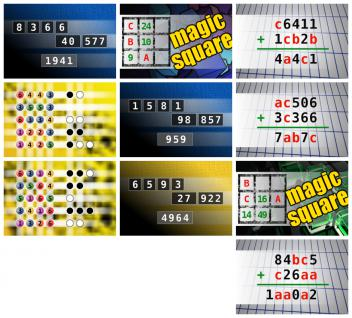Daily Brain Teasers for Friday, 29 September 2023
● NUMBERMANIA: Calculate the number 1941 using numbers [8, 3, 6, 6, 40, 577] and basic arithmetic operations (+, -, *, /). Each of the numbers can be used only once. ● The aim is to place the some numbers from the list (9, 10, 13, 14, 15, 18, 24, 25, 28, 62) into the empty squares and squares marked with A, B an C. Sum of each row and column should be equal. All the numbers of the magic square must be different. Find values for A, B, and C. Solution is A+B+C. ● If c6411 + 1cb2b = 4a4c1 find number abc. Multiple solutions may exist. ● The computer chose a secret code (sequence of 4 digits from 1 to 6). Your goal is to find that code. Black circles indicate the number of hits on the right spot. White circles indicate the number of hits on the wrong spot. ● NUMBERMANIA: Calculate the number 959 using numbers [1, 5, 8, 1, 98, 857] and basic arithmetic operations (+, -, *, /). Each of the numbers can be used only once. ● If ac506 + 3c366 = 7ab7c find number abc. Multiple solutions may exist. ● The computer chose a secret code (sequence of 4 digits from 1 to 6). Your goal is to find that code. Black circles indicate the number of hits on the right spot. White circles indicate the number of hits on the wrong spot. ● NUMBERMANIA: Calculate the number 4964 using numbers [6, 5, 9, 3, 27, 922] and basic arithmetic operations (+, -, *, /). Each of the numbers can be used only once. ● The aim is to place the some numbers from the list (13, 14, 16, 20, 21, 23, 42, 49, 50, 52, 58, 79) into the empty squares and squares marked with A, B an C. Sum of each row and column should be equal. All the numbers of the magic square must be different. Find values for A, B, and C. Solution is A*B+C. ● If 84bc5 + c26aa = 1aa0a2 find number abc. Multiple solutions may exist.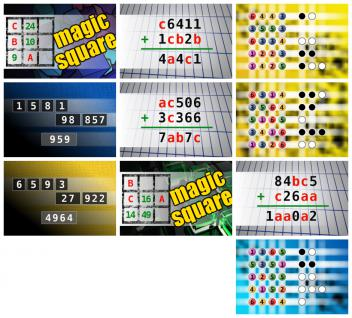Daily Brain Teasers for Thursday, 28 September 2023
● The aim is to place the some numbers from the list (9, 10, 13, 14, 15, 18, 24, 25, 28, 62) into the empty squares and squares marked with A, B an C. Sum of each row and column should be equal. All the numbers of the magic square must be different. Find values for A, B, and C. Solution is A+B+C. ● If c6411 + 1cb2b = 4a4c1 find number abc. Multiple solutions may exist. ● The computer chose a secret code (sequence of 4 digits from 1 to 6). Your goal is to find that code. Black circles indicate the number of hits on the right spot. White circles indicate the number of hits on the wrong spot. ● NUMBERMANIA: Calculate the number 959 using numbers [1, 5, 8, 1, 98, 857] and basic arithmetic operations (+, -, *, /). Each of the numbers can be used only once. ● If ac506 + 3c366 = 7ab7c find number abc. Multiple solutions may exist. ● The computer chose a secret code (sequence of 4 digits from 1 to 6). Your goal is to find that code. Black circles indicate the number of hits on the right spot. White circles indicate the number of hits on the wrong spot. ● NUMBERMANIA: Calculate the number 4964 using numbers [6, 5, 9, 3, 27, 922] and basic arithmetic operations (+, -, *, /). Each of the numbers can be used only once. ● The aim is to place the some numbers from the list (13, 14, 16, 20, 21, 23, 42, 49, 50, 52, 58, 79) into the empty squares and squares marked with A, B an C. Sum of each row and column should be equal. All the numbers of the magic square must be different. Find values for A, B, and C. Solution is A*B+C. ● If 84bc5 + c26aa = 1aa0a2 find number abc. Multiple solutions may exist. ● The computer chose a secret code (sequence of 4 digits from 1 to 6). Your goal is to find that code. Black circles indicate the number of hits on the right spot. White circles indicate the number of hits on the wrong spot.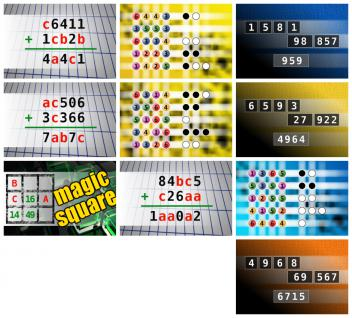Daily Brain Teasers for Wednesday, 27 September 2023
● If c6411 + 1cb2b = 4a4c1 find number abc. Multiple solutions may exist. ● The computer chose a secret code (sequence of 4 digits from 1 to 6). Your goal is to find that code. Black circles indicate the number of hits on the right spot. White circles indicate the number of hits on the wrong spot. ● NUMBERMANIA: Calculate the number 959 using numbers [1, 5, 8, 1, 98, 857] and basic arithmetic operations (+, -, *, /). Each of the numbers can be used only once. ● If ac506 + 3c366 = 7ab7c find number abc. Multiple solutions may exist. ● The computer chose a secret code (sequence of 4 digits from 1 to 6). Your goal is to find that code. Black circles indicate the number of hits on the right spot. White circles indicate the number of hits on the wrong spot. ● NUMBERMANIA: Calculate the number 4964 using numbers [6, 5, 9, 3, 27, 922] and basic arithmetic operations (+, -, *, /). Each of the numbers can be used only once. ● The aim is to place the some numbers from the list (13, 14, 16, 20, 21, 23, 42, 49, 50, 52, 58, 79) into the empty squares and squares marked with A, B an C. Sum of each row and column should be equal. All the numbers of the magic square must be different. Find values for A, B, and C. Solution is A*B+C. ● If 84bc5 + c26aa = 1aa0a2 find number abc. Multiple solutions may exist. ● The computer chose a secret code (sequence of 4 digits from 1 to 6). Your goal is to find that code. Black circles indicate the number of hits on the right spot. White circles indicate the number of hits on the wrong spot. ● NUMBERMANIA: Calculate the number 6715 using numbers [4, 9, 6, 8, 69, 567] and basic arithmetic operations (+, -, *, /). Each of the numbers can be used only once.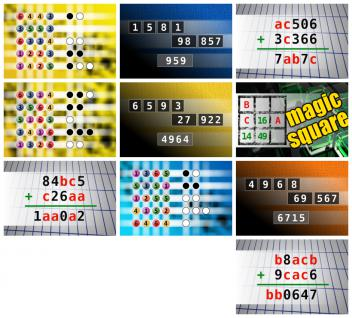Daily Brain Teasers for Tuesday, 26 September 2023
● The computer chose a secret code (sequence of 4 digits from 1 to 6). Your goal is to find that code. Black circles indicate the number of hits on the right spot. White circles indicate the number of hits on the wrong spot. ● NUMBERMANIA: Calculate the number 959 using numbers [1, 5, 8, 1, 98, 857] and basic arithmetic operations (+, -, *, /). Each of the numbers can be used only once. ● If ac506 + 3c366 = 7ab7c find number abc. Multiple solutions may exist. ● The computer chose a secret code (sequence of 4 digits from 1 to 6). Your goal is to find that code. Black circles indicate the number of hits on the right spot. White circles indicate the number of hits on the wrong spot. ● NUMBERMANIA: Calculate the number 4964 using numbers [6, 5, 9, 3, 27, 922] and basic arithmetic operations (+, -, *, /). Each of the numbers can be used only once. ● The aim is to place the some numbers from the list (13, 14, 16, 20, 21, 23, 42, 49, 50, 52, 58, 79) into the empty squares and squares marked with A, B an C. Sum of each row and column should be equal. All the numbers of the magic square must be different. Find values for A, B, and C. Solution is A*B+C. ● If 84bc5 + c26aa = 1aa0a2 find number abc. Multiple solutions may exist. ● The computer chose a secret code (sequence of 4 digits from 1 to 6). Your goal is to find that code. Black circles indicate the number of hits on the right spot. White circles indicate the number of hits on the wrong spot. ● NUMBERMANIA: Calculate the number 6715 using numbers [4, 9, 6, 8, 69, 567] and basic arithmetic operations (+, -, *, /). Each of the numbers can be used only once. ● If b8acb + 9cac6 = bb0647 find number abc. Multiple solutions may exist.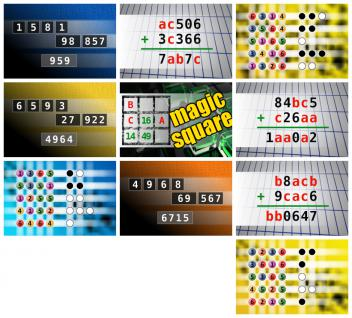Daily Brain Teasers for Monday, 25 September 2023
● NUMBERMANIA: Calculate the number 959 using numbers [1, 5, 8, 1, 98, 857] and basic arithmetic operations (+, -, *, /). Each of the numbers can be used only once. ● If ac506 + 3c366 = 7ab7c find number abc. Multiple solutions may exist. ● The computer chose a secret code (sequence of 4 digits from 1 to 6). Your goal is to find that code. Black circles indicate the number of hits on the right spot. White circles indicate the number of hits on the wrong spot. ● NUMBERMANIA: Calculate the number 4964 using numbers [6, 5, 9, 3, 27, 922] and basic arithmetic operations (+, -, *, /). Each of the numbers can be used only once. ● The aim is to place the some numbers from the list (13, 14, 16, 20, 21, 23, 42, 49, 50, 52, 58, 79) into the empty squares and squares marked with A, B an C. Sum of each row and column should be equal. All the numbers of the magic square must be different. Find values for A, B, and C. Solution is A*B+C. ● If 84bc5 + c26aa = 1aa0a2 find number abc. Multiple solutions may exist. ● The computer chose a secret code (sequence of 4 digits from 1 to 6). Your goal is to find that code. Black circles indicate the number of hits on the right spot. White circles indicate the number of hits on the wrong spot. ● NUMBERMANIA: Calculate the number 6715 using numbers [4, 9, 6, 8, 69, 567] and basic arithmetic operations (+, -, *, /). Each of the numbers can be used only once. ● If b8acb + 9cac6 = bb0647 find number abc. Multiple solutions may exist. ● The computer chose a secret code (sequence of 4 digits from 1 to 6). Your goal is to find that code. Black circles indicate the number of hits on the right spot. White circles indicate the number of hits on the wrong spot.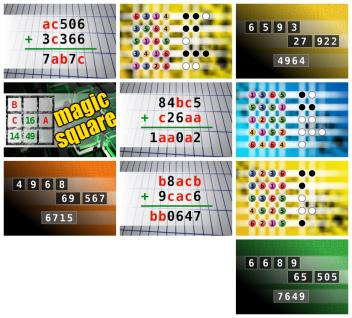Daily Brain Teasers for Sunday, 24 September 2023
● If ac506 + 3c366 = 7ab7c find number abc. Multiple solutions may exist. ● The computer chose a secret code (sequence of 4 digits from 1 to 6). Your goal is to find that code. Black circles indicate the number of hits on the right spot. White circles indicate the number of hits on the wrong spot. ● NUMBERMANIA: Calculate the number 4964 using numbers [6, 5, 9, 3, 27, 922] and basic arithmetic operations (+, -, *, /). Each of the numbers can be used only once. ● The aim is to place the some numbers from the list (13, 14, 16, 20, 21, 23, 42, 49, 50, 52, 58, 79) into the empty squares and squares marked with A, B an C. Sum of each row and column should be equal. All the numbers of the magic square must be different. Find values for A, B, and C. Solution is A*B+C. ● If 84bc5 + c26aa = 1aa0a2 find number abc. Multiple solutions may exist. ● The computer chose a secret code (sequence of 4 digits from 1 to 6). Your goal is to find that code. Black circles indicate the number of hits on the right spot. White circles indicate the number of hits on the wrong spot. ● NUMBERMANIA: Calculate the number 6715 using numbers [4, 9, 6, 8, 69, 567] and basic arithmetic operations (+, -, *, /). Each of the numbers can be used only once. ● If b8acb + 9cac6 = bb0647 find number abc. Multiple solutions may exist. ● The computer chose a secret code (sequence of 4 digits from 1 to 6). Your goal is to find that code. Black circles indicate the number of hits on the right spot. White circles indicate the number of hits on the wrong spot. ● NUMBERMANIA: Calculate the number 7649 using numbers [6, 6, 8, 9, 65, 505] and basic arithmetic operations (+, -, *, /). Each of the numbers can be used only once.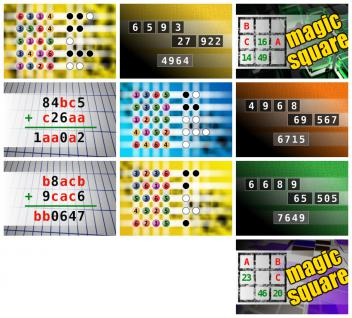Daily Brain Teasers for Saturday, 23 September 2023
● The computer chose a secret code (sequence of 4 digits from 1 to 6). Your goal is to find that code. Black circles indicate the number of hits on the right spot. White circles indicate the number of hits on the wrong spot. ● NUMBERMANIA: Calculate the number 4964 using numbers [6, 5, 9, 3, 27, 922] and basic arithmetic operations (+, -, *, /). Each of the numbers can be used only once. ● The aim is to place the some numbers from the list (13, 14, 16, 20, 21, 23, 42, 49, 50, 52, 58, 79) into the empty squares and squares marked with A, B an C. Sum of each row and column should be equal. All the numbers of the magic square must be different. Find values for A, B, and C. Solution is A*B+C. ● If 84bc5 + c26aa = 1aa0a2 find number abc. Multiple solutions may exist. ● The computer chose a secret code (sequence of 4 digits from 1 to 6). Your goal is to find that code. Black circles indicate the number of hits on the right spot. White circles indicate the number of hits on the wrong spot. ● NUMBERMANIA: Calculate the number 6715 using numbers [4, 9, 6, 8, 69, 567] and basic arithmetic operations (+, -, *, /). Each of the numbers can be used only once. ● If b8acb + 9cac6 = bb0647 find number abc. Multiple solutions may exist. ● The computer chose a secret code (sequence of 4 digits from 1 to 6). Your goal is to find that code. Black circles indicate the number of hits on the right spot. White circles indicate the number of hits on the wrong spot. ● NUMBERMANIA: Calculate the number 7649 using numbers [6, 6, 8, 9, 65, 505] and basic arithmetic operations (+, -, *, /). Each of the numbers can be used only once. ● The aim is to place the some numbers from the list (1, 18, 20, 23, 27, 29, 32, 39, 41, 43, 46, 74) into the empty squares and squares marked with A, B an C. Sum of each row and column should be equal. All the numbers of the magic square must be different. Find values for A, B, and C. Solution is A-B+C.
 Follow Brain Teasers on social networks

## Top 10 Users (1174)

 1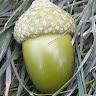H Tav 5861 2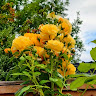c. raj. 5777 3Nasrin 24 T 5731 4Fazil Hashim 5718 5Thinh Ddh 5557 6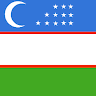Alfa Omega 4681 7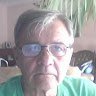Djordje Timotijevic 4549 8Mita Kojd 4525 9Jakubovski Vladimir 4443 10Chandu Rajyaguru 4419
See full ranking list

### Atomic bomb security

In 1945, following a message from President Truman, a bill sponsored by the war department and known as the May-Johnson bill was introduced into the U.S. Congress. The purpose of this bill was to keep the atomic bomb a secret under stringent security restrictions. Because it failed to provide for the sharing of information with foreign countries, and granted a dominant role to the military, scientists throughout the country were galvanized in opposition. Due in part to lobbying by scientists such as Leo Szilard and other groups, the May-Johnson Bill was tabled in December. The McMahon Act, signed on 1 Aug 1946, mandated civilian control of atomic energy under the auspices of the Atomic Energy Commission (AEC).
This site uses cookies to store information on your computer. Some are essential to help the site properly. Others give us insight into how the site is used and help us to optimize the user experience. See our privacy policy.